### IMO Shortlist 2000 problem C3

Kvaliteta:
Avg: 0,0
Težina:
Avg: 7,0
Let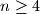$n \geq 4$ be a fixed positive integer. Given a set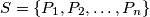$S = \{P_1, P_2, \ldots, P_n\}$ of$n$ points in the plane such that no three are collinear and no four concyclic, let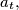$a_t,$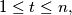$1 \leq t \leq n,$ be the number of circles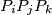$P_iP_jP_k$ that contain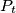$P_t$ in their interior, and let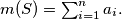$m(S) = \sum^n_{i=1} a_i.$ Prove that there exists a positive integer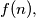$f(n),$ depending only on$n,$ such that the points of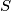$S$ are the vertices of a convex polygon if and only if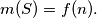$m(S) = f(n).$
Izvor: Međunarodna matematička olimpijada, shortlist 2000